Balbharti Maharashtra Board Class 5 Maths Solutions Chapter 5 Fractions Problem Set 21 Textbook Exercise Important Questions and Answers.

## Maharashtra State Board Class 5 Maths Solutions Chapter 5 Fractions Problem Set 21

Question 1.
Subtract the following.

$$\text { (1) } \frac{5}{7}-\frac{1}{7}$$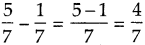$$\text { (2) } \frac{5}{8}-\frac{3}{8}$$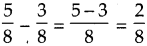$$\text { (3) } \frac{7}{9}-\frac{2}{9}$$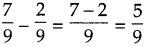$$\text { (4) } \frac{8}{11}-\frac{5}{11}$$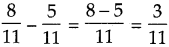$$\text { (5) } \frac{9}{13}-\frac{4}{13}$$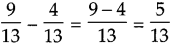$$\text { (6) } \frac{7}{10}-\frac{3}{10}$$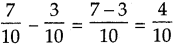$$\text { (7) } \frac{9}{12}-\frac{2}{12}$$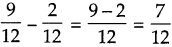$$\text { (8) } \frac{10}{15}-\frac{3}{15}$$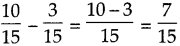Question 2.
$$\frac{7}{10}$$ of a wall is to be painted. Ramu has painted 410 of it. How much more needs to be painted?
Solution:
To be painted – painted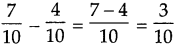$$\frac{3}{10}$$ more needs to be painted.

Addition and subtraction of unlike fractions

Example (1) Add : $$\frac{2}{3}+\frac{1}{6}$$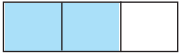First let us show the fraction $$\frac{2}{3}$$ by coloring two of the three equal parts on a strip.

You have learnt to add and to subtract fractions with common denominators. Here, we have to add the fraction $$\frac{1}{6}$$ to the fraction $$\frac{2}{3}$$.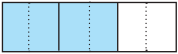So let us divide each part on this strip into two equal parts. $$\frac{4}{6}$$ is a fraction equivalent to $$\frac{2}{3}$$. Now, as 16 is to be added to $$\frac{2}{3}$$ i.e. to $$\frac{4}{6}$$, we shall colour one more of the six parts on the strip. Now, the total coloured part is $$\frac{5}{6}$$.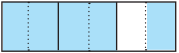Therefore,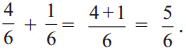That is,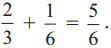Example (2) Add : $$\frac{1}{2}+\frac{2}{5}$$
Here, the smallest common multiple of the two denominators is 10. So, we shall change the denominator of both fractions to 10.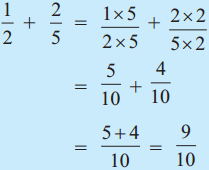Example (3) Add : $$\frac{3}{8}+\frac{1}{16}$$
Here, 16 is twice 8. So, we shall change the denominator of both fractions to 16.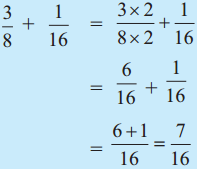Example (4) Subtract : $$\frac{3}{4}-\frac{5}{8}$$
Let us make 8 the common denominator of the given fractions.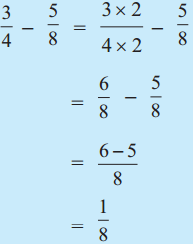Example (5) Subtract : $$\frac{4}{5}-\frac{2}{3}$$
The smallest common multiple of the denominators is 15. So, we shall change the denominator of both fractions to 15.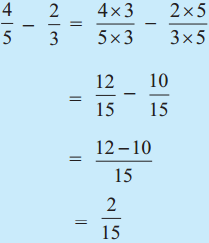$$\text { (1) } \frac{9}{14}-\frac{3}{14}$$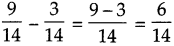$$\text { (2) } \frac{5}{6}-\frac{3}{6}$$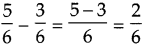$$\text { (3) } \frac{9}{16}-\frac{5}{16}$$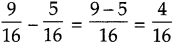$$\text { (4) } \frac{7}{8}-\frac{3}{8}-\frac{1}{8}$$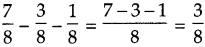(5) $$\frac{7}{9}$$ part of the work done by Neha and Supriya together. $$\frac{5}{9}$$ part of this work was done by Neha. How much work done by Supriya?
Solution:
Total work done – work done by Neha = work done by Supriya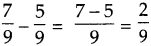$$\frac{2}{9}$$ work done by Supriya(6) Mr. Sharma is $$\frac{14}{9}$$ m tall. Mrs. Sharma is $$\frac{4}{9}$$ shorter than him. What is Mrs. Sharma’s height?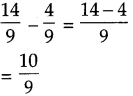Mrs. Sharma’s height = $$\frac{10}{9}$$